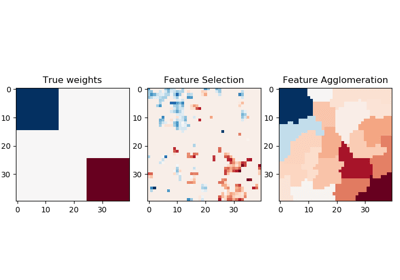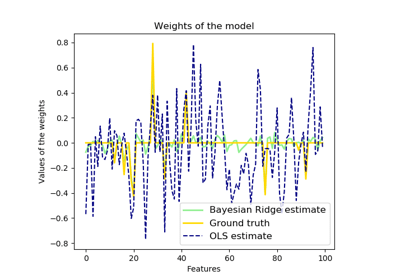/scikit-learn

# sklearn.linear_model.BayesianRidge

`class sklearn.linear_model.BayesianRidge(n_iter=300, tol=0.001, alpha_1=1e-06, alpha_2=1e-06, lambda_1=1e-06, lambda_2=1e-06, compute_score=False, fit_intercept=True, normalize=False, copy_X=True, verbose=False)` [source]

Bayesian ridge regression

Fit a Bayesian ridge model and optimize the regularization parameters lambda (precision of the weights) and alpha (precision of the noise).

Read more in the User Guide.

Parameters: `n_iter : int, optional` Maximum number of iterations. Default is 300. `tol : float, optional` Stop the algorithm if w has converged. Default is 1.e-3. `alpha_1 : float, optional` Hyper-parameter : shape parameter for the Gamma distribution prior over the alpha parameter. Default is 1.e-6 `alpha_2 : float, optional` Hyper-parameter : inverse scale parameter (rate parameter) for the Gamma distribution prior over the alpha parameter. Default is 1.e-6. `lambda_1 : float, optional` Hyper-parameter : shape parameter for the Gamma distribution prior over the lambda parameter. Default is 1.e-6. `lambda_2 : float, optional` Hyper-parameter : inverse scale parameter (rate parameter) for the Gamma distribution prior over the lambda parameter. Default is 1.e-6 `compute_score : boolean, optional` If True, compute the objective function at each step of the model. Default is False `fit_intercept : boolean, optional` whether to calculate the intercept for this model. If set to false, no intercept will be used in calculations (e.g. data is expected to be already centered). Default is True. `normalize : boolean, optional, default False` This parameter is ignored when `fit_intercept` is set to False. If True, the regressors X will be normalized before regression by subtracting the mean and dividing by the l2-norm. If you wish to standardize, please use `sklearn.preprocessing.StandardScaler` before calling `fit` on an estimator with `normalize=False`. `copy_X : boolean, optional, default True` If True, X will be copied; else, it may be overwritten. `verbose : boolean, optional, default False` Verbose mode when fitting the model. `coef_ : array, shape = (n_features)` Coefficients of the regression model (mean of distribution) `alpha_ : float` estimated precision of the noise. `lambda_ : float` estimated precision of the weights. `sigma_ : array, shape = (n_features, n_features)` estimated variance-covariance matrix of the weights `scores_ : float` if computed, value of the objective function (to be maximized)

#### Notes

For an example, see examples/linear_model/plot_bayesian_ridge.py.

#### References

D. J. C. MacKay, Bayesian Interpolation, Computation and Neural Systems, Vol. 4, No. 3, 1992.

R. Salakhutdinov, Lecture notes on Statistical Machine Learning, http://www.utstat.toronto.edu/~rsalakhu/sta4273/notes/Lecture2.pdf#page=15 Their beta is our `self.alpha_` Their alpha is our `self.lambda_`

#### Examples

```>>> from sklearn import linear_model
>>> clf = linear_model.BayesianRidge()
>>> clf.fit([[0,0], [1, 1], [2, 2]], [0, 1, 2])
...
BayesianRidge(alpha_1=1e-06, alpha_2=1e-06, compute_score=False,
copy_X=True, fit_intercept=True, lambda_1=1e-06, lambda_2=1e-06,
n_iter=300, normalize=False, tol=0.001, verbose=False)
>>> clf.predict([[1, 1]])
array([1.])
```

#### Methods

 `fit`(X, y[, sample_weight]) Fit the model `get_params`([deep]) Get parameters for this estimator. `predict`(X[, return_std]) Predict using the linear model. `score`(X, y[, sample_weight]) Returns the coefficient of determination R^2 of the prediction. `set_params`(**params) Set the parameters of this estimator.
`__init__(n_iter=300, tol=0.001, alpha_1=1e-06, alpha_2=1e-06, lambda_1=1e-06, lambda_2=1e-06, compute_score=False, fit_intercept=True, normalize=False, copy_X=True, verbose=False)` [source]
`fit(X, y, sample_weight=None)` [source]

Fit the model

Parameters: `X : numpy array of shape [n_samples,n_features]` Training data `y : numpy array of shape [n_samples]` Target values. Will be cast to X’s dtype if necessary `sample_weight : numpy array of shape [n_samples]` Individual weights for each sample New in version 0.20: parameter sample_weight support to BayesianRidge. `self : returns an instance of self.`
`get_params(deep=True)` [source]

Get parameters for this estimator.

Parameters: `deep : boolean, optional` If True, will return the parameters for this estimator and contained subobjects that are estimators. `params : mapping of string to any` Parameter names mapped to their values.
`predict(X, return_std=False)` [source]

Predict using the linear model.

In addition to the mean of the predictive distribution, also its standard deviation can be returned.

Parameters: `X : {array-like, sparse matrix}, shape = (n_samples, n_features)` Samples. `return_std : boolean, optional` Whether to return the standard deviation of posterior prediction. `y_mean : array, shape = (n_samples,)` Mean of predictive distribution of query points. `y_std : array, shape = (n_samples,)` Standard deviation of predictive distribution of query points.
`score(X, y, sample_weight=None)` [source]

Returns the coefficient of determination R^2 of the prediction.

The coefficient R^2 is defined as (1 - u/v), where u is the residual sum of squares ((y_true - y_pred) ** 2).sum() and v is the total sum of squares ((y_true - y_true.mean()) ** 2).sum(). The best possible score is 1.0 and it can be negative (because the model can be arbitrarily worse). A constant model that always predicts the expected value of y, disregarding the input features, would get a R^2 score of 0.0.

Parameters: `X : array-like, shape = (n_samples, n_features)` Test samples. For some estimators this may be a precomputed kernel matrix instead, shape = (n_samples, n_samples_fitted], where n_samples_fitted is the number of samples used in the fitting for the estimator. `y : array-like, shape = (n_samples) or (n_samples, n_outputs)` True values for X. `sample_weight : array-like, shape = [n_samples], optional` Sample weights. `score : float` R^2 of self.predict(X) wrt. y.
`set_params(**params)` [source]

Set the parameters of this estimator.

The method works on simple estimators as well as on nested objects (such as pipelines). The latter have parameters of the form `<component>__<parameter>` so that it’s possible to update each component of a nested object.

Returns: self

## Examples using `sklearn.linear_model.BayesianRidge`Feature agglomeration vs. univariate selectionBayesian Ridge Regression